# How to use nested IF formulas in Excel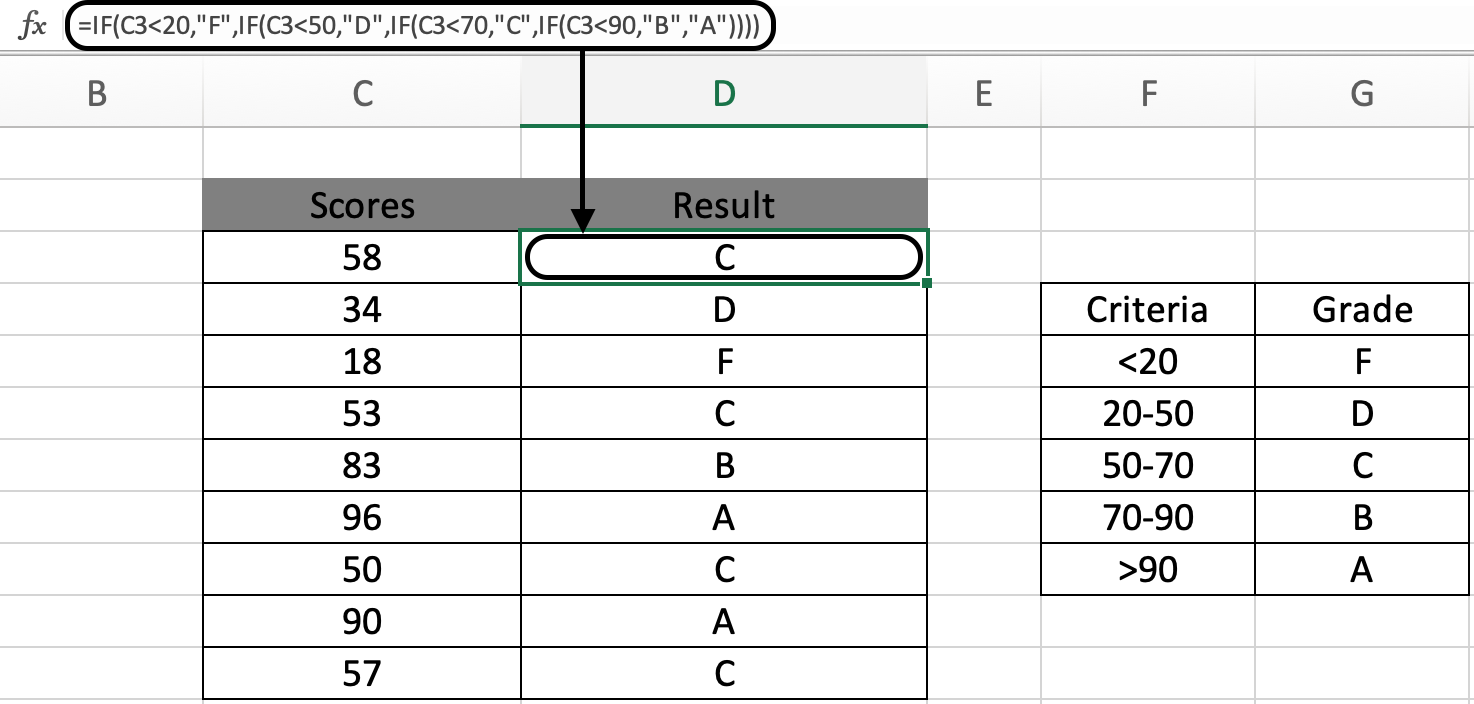In this article, we will learn How to use nested IF formulas in Excel.

What are nested formulas in Excel ?

Nest means a pile of things built of multiple single things. When you start doing excel you will not evaluate the result using one function at a time. You use multiple functions in one formula. This is done to save space and time and is usual practice. Let's learn nested formula syntax and illustrate an example to understand better.

nested IF formula Function in Excel

IF function is used for logic_test and returns value on the basis of the result of the logic_test.

Formula Syntax:

 =IF(first_condition ,value_if_first_true, IF(second_condition, value_if_second_true, third_condition, value_if_third_true, value_if_none_is_true)

Nested IF is an iteration of IF function. It runs multiple logic_test in one formula. IFS function is built in function use instead for nested IF function in version above Excel 2016..

Example :

All of these might be confusing to understand. Let's understand how to use the function using an example. Here we gave a list of student scored marks on a test. As a teacher you need to update the grade column evaluating the scores criteria.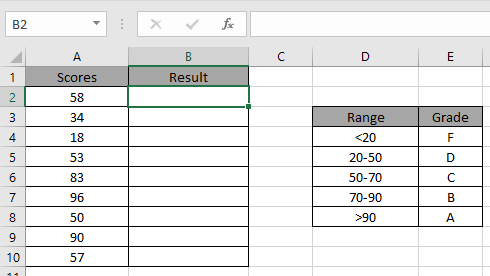We will use the below process. Let's understand.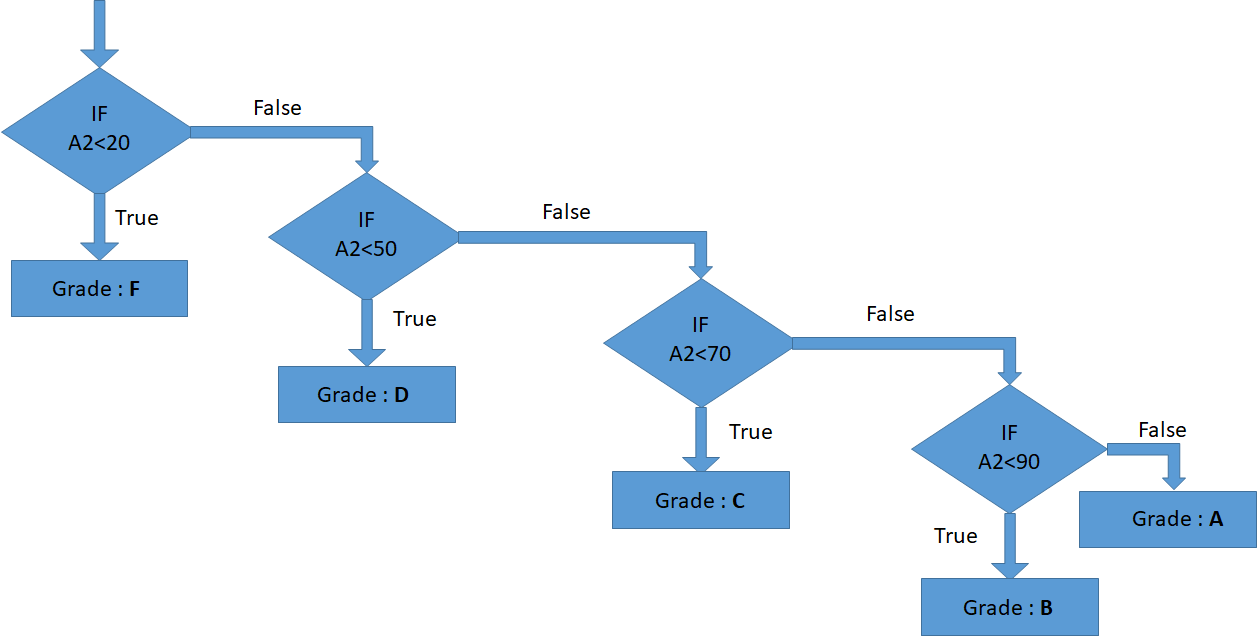Use the formula:

 =IF(C3 < 20,"F",IF(C3 < 50,"D",IF(C3 < 70,"C",IF(C3 < 90,"B","A"))))

C3<20 : test1

“F”: return F if test1 satisfies.

C3<50: test2

“D”: return D if test2 satisfies.

C3<90: test3

”B”: return B if test3 satisfies.

C3>90: test4

“A”: return A if test3 satisfies.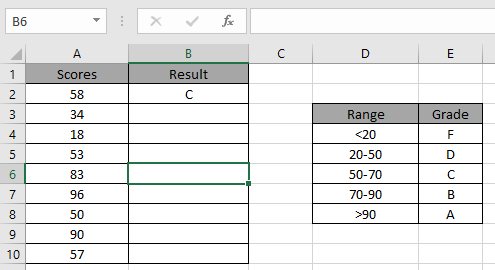Applying the formula in other cells using CTRL + D.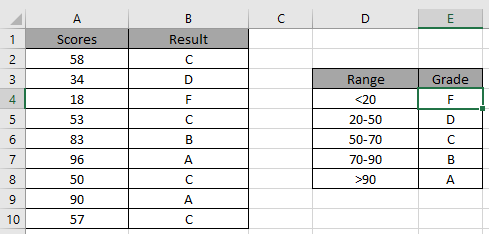As you can see it’s easy to get the Grades having multiple conditions.

IF - AND Function in Excel

Here We have a list of Scores and We need to know under which criteria it lies.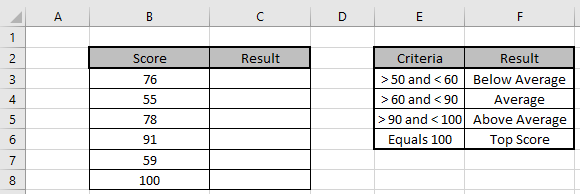Here multiple criterias are needed to satisfy. Use the formula to match the given criteria table

Formula:

 =IF(AND(B3 > 50, B3 < 60), "Below Average",IF(AND(B3 > 60, B3 < 90),"Average",IF(AND(B3 > 90,B3< 100),"Above Average","Top Score")))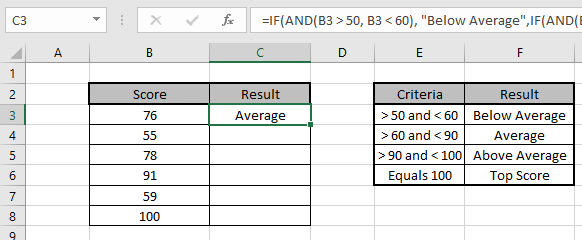Copy the formula in other cells, select the cells taking the first cell where the formula is already applied, use shortcut key Ctrl + D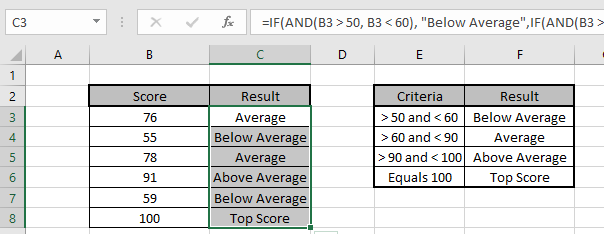We got the Results corresponding to the Scores.

You can use IF and AND function to meet multiple conditions in a single formula.

Here are all the observational notes using the nested IF formula in Excel
Notes :

1. Use the IFS function in the latest version of excel (after  Excel 2017).
2. Logic Operators like equals to ( = ), less than equal to ( <= ), greater than ( > ) or not equals to ( <> ) can be performed within a function applied with number only.
3. Mathematical operators like SUM ( + ), Subtract ( - ), product ( * ), division ( / ), percent ( % ), power ( ^ ) can be performed over numbers using the example.

Hope this article about How to use nested IF formulas in Excel is explanatory. Find more articles on calculating values and related Excel formulas here. If you liked our blogs, share it with your friends on Facebook. And also you can follow us on Twitter and Facebook. We would love to hear from you, do let us know how we can improve, complement or innovate our work and make it better for you. Write to us at info@exceltip.com.

Related Articles :

IF with AND function : Implementation of logic IF function with AND function to extract results having criteria in Excel.

IF with OR function : Implementation of logic IF function with OR function to extract results having criteria in excel data.

How to use the IFS function : nested IF function operates on data having multiple criteria. The use of repeated IF function is nested IF excel formula.

SUMIFS using AND-OR logic : Get the sum of numbers having multiple criteria applied using logic AND-OR excel function.

Minimum value using IF function : Get the minimum value using the excel IF function and MIN function on array data.

How to use wildcards in excel : Count cells matching phrases using the wildcards in excel

Popular Articles :

How to use the IF Function in Excel : The IF statement in Excel checks the condition and returns a specific value if the condition is TRUE or returns another specific value if FALSE.

How to use the VLOOKUP Function in Excel : This is one of the most used and popular functions of excel that is used to lookup value from different ranges and sheets.

How to use the SUMIF Function in Excel : This is another dashboard essential function. This helps you sum up values on specific conditions.

How to use the COUNTIF Function in Excel : Count values with conditions using this amazing function. You don't need to filter your data to count specific values. Countif function is essential to prepare your dashboard.

Terms and Conditions of use

The applications/code on this site are distributed as is and without warranties or liability. In no event shall the owner of the copyrights, or the authors of the applications/code be liable for any loss of profit, any problems or any damage resulting from the use or evaluation of the applications/code.